output.to from Sideway
Mechanics: StaticsMechanics Dynamics

System of ForcesSystem of Forces TransformationEquivalent systems of forces

Draft for Information Only

# Content

`System of Forces Transformation Force Couple Force Vector`

# System of Forces Transformation

Sometimes a system of forces acting on a rigid body is needed to be transformed into an equivalent system at the point of interest.

## Force Couple

A couple of force F is applied at points A, B on a rigid body.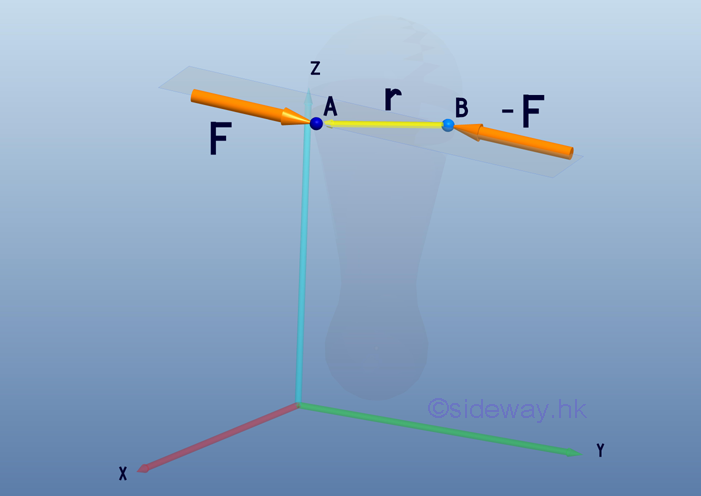The equivalent system is a free couple vector M acting on any location of the rigid body.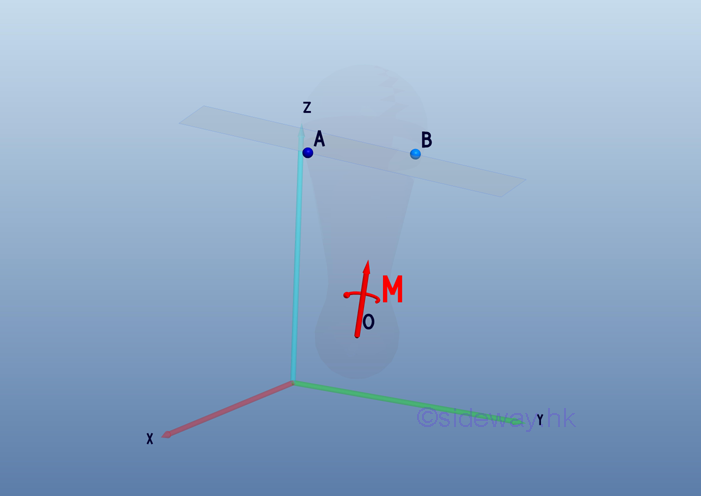Consider the moment MBA at point B,  the tendency of rotation above B is caused by the applied force F at A. The applied force -F at B can be considered as the reaction supporting force. Both the magnitude and direction of the moment vector MBA  is same as the couple vector M.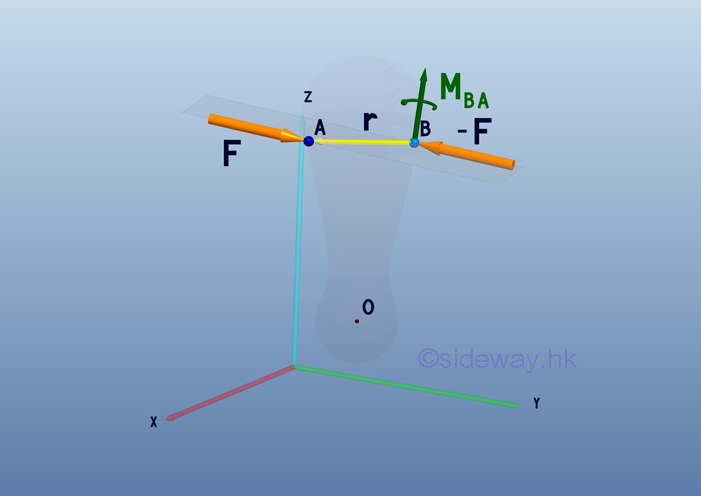## Force Vector

Suppose a force vector acting at point A on the rigid body is to be considered at point B.When only a single force F is applied at point A on the rigid body, the moment of the force at point A about point B is also equal to  MBA .To simulate the force couple condition, two forces, F and  -F with magnitude equal to the applied force F are attached at point B. After this transformation, a force couple of moment MBA is formed with an additional force -F acting at point B.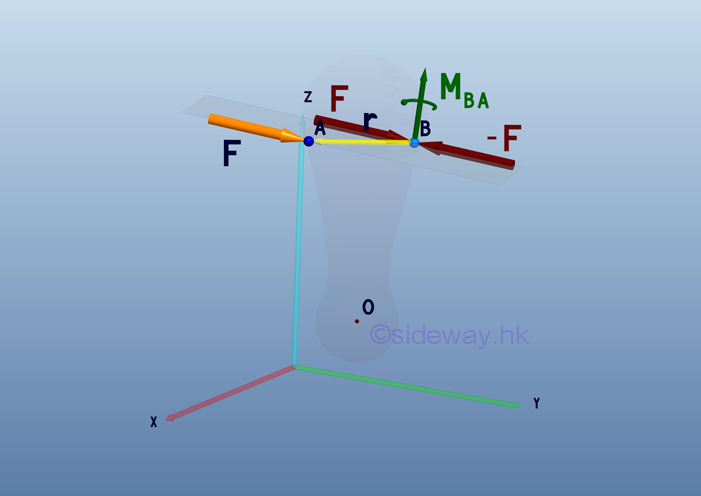Force F acting at point A is now moved to point B. The formed force couple of moment MBA can be replaced by a couple vector M. The couple vector M is a free vector and it can be located at point B for convenience.Therefore the equivalent system of a force vector F acting at point A on a rigid body can be transformed to an arbitrary point B by moving the force vector to pont B and adding a couple vector M with moment equals to the moment MBA of the force vector F about point B. At which, the force vector F tend to provide the same linear motion and the couple vector M tend to provide the same rotational motion as the force vector F at point A. The equivalent system of combining a force vector and a couple vector is named as force-couple system. And the couple vector is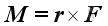ID: 110700010 Last Updated: 12/7/2011 Revision: 1 Ref:References

1. F.P. Beer; E.R. Johnston,Jr.; E.R. Eisenberg, 2004, Vector Mechanics for Engineers: Statics
2. I.C. Jong; B.G. rogers, 1991, Engineering Mechanics: Statics and DynamicsHome 5

Management

HBR 3

Information

Recreation

Culture

Chinese 1097

English 337

Computer

Hardware 151

Software

Application 202

Manim 123

Numeric 19

Programming

Web 285

Unicode 494

HTML 65

CSS 59

ASP.NET 194

OS 391

Python 23

Knowledge

Mathematics

Algebra 29

Geometry 21

Calculus 67

Engineering

Mechanical

Rigid Bodies

Statics 92

Dynamics 37

Control

Physics

Electric 27